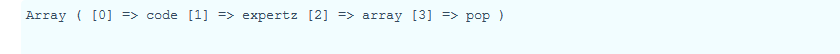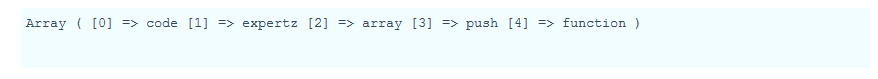# Array pop and Array push functions in php with examples

Array pop() function is used to delete the last element in an array

Array push() function is used to insert the element in the array

Array pop

Syntax:

array_pop(\$array);

\$array is variable in which the values are stored

Example:

```\$array_pop_variable=array("code","expertz","array","pop","function");
array_pop(\$array_pop_variable);
print_r(\$array_pop_variable); ```

Output:Array push

Syntax:

array_push(\$array ,value1 ,value2);

\$array is variable in which the values are stored.

Value1 and value2  are the datas which needs to be inserted into the array contained in variable  \$array.

Example:

```\$array_push_variable = array("code", "expertz");
array_push(\$array_push_variable, "array", "push" ,"function");
print_r(\$array_push_variable); ```

Output:Category: